Signal Processing
Fourier Transformation - Solution

Seismo-Live: http://seismo-live.org

##### Authors:¶

Some code used in this tutorial is taken from stackoverflow.com. We thank Giulio Ghirardo for his kind permission to use his code here.

# Tutorial on Fourier transformation in 1D

In :
# Cell 0 - Preparation: load packages, set some basic options
%matplotlib inline
from scipy import signal
from obspy.signal.invsim import cosine_taper
from matplotlib import rcParams
import numpy as np
import matplotlib.pylab as plt
plt.style.use('ggplot')
plt.rcParams['figure.figsize'] = 15, 3


## The Fourier transformation¶

In the world of seismology, we use the Fourier transformation to transform a signal from the time domain into the frequency domain. That means, we split up the signal and separate the content of each frequency from each other. Doing so, we can analyse our signal according to energy content per frequency. We can extract information on how much amplitude each frequency contributes to the final signal. In other words: we get a receipt of the ingredients we need to blend our measured signal.

The Fourier transformation is based on the Fourier series. With the Fourier series we can approximate an (unknown) function $f(x)$ by another function $g_n(x)$ which consists of a sum over $N$ basis functions weighted by some coefficients. The basis functions need to be orthogonal. $sin$ and $cos$ functions seem to be a pretty good choice because any signal can be filtered into several sinusoidal paths. In the period range of $[-T/2 ; T/2]$ the Fourier series is defined as:

$$f(t) \approx g_n(t) = \frac{1}{2} a_0 + \sum_{k=1}^N \left[ a_k \cos \left(\frac{2\pi k t}{T} \right) + b_k \sin\left(\frac{2\pi k t}{T}\right)\right]$$$$a_k = \frac{2}{T} \int_{-T/2}^{T/2} f(t) \cos\left(\frac{2\pi k t}{T}\right)dt$$$$b_k = \frac{2}{T} \int_{-T/2}^{T/2} f(t) \sin\left(\frac{2\pi k t}{T}\right)dt$$

At this stage, we consider continuous, periodic and infinite functions. The more basis functions are used to approximate the unknown function, the better is the approximation, i.e. the more similar the unknown function is to its approximation.

For a non-periodic function the interval of periodicity tends to infinity. That means, the steps between neighbouring frequencies become smaller and smaller and thus the infinite sum of the Fourier series turns into an integral and we end up with the integral form of the Fourier transformation:

$$F(\omega) = \frac{1}{2\pi} \int_{-\infty}^{\infty} f(t) e^{-i\omega t} dt \leftrightarrow f(t) = \int_{-\infty}^{\infty} F(\omega)e^{i\omega t}dt$$

Attention: sign and factor conventions can be different in the literature!

In seismology, we do not have continuous but discrete time signals. Therefore, we work with the discrete form of the Fourier transformation:

$$F_k = \frac{1}{N} \sum_{j=0}^{N-1} f_j e^{-2\pi i k j /N} \leftrightarrow f_k = \sum_{j=0}^{N-1} F_j e^{2\pi i k j /N}$$

Some intuitive gif animations on what the Fourier transform is doing, can be found here, here, and here. Further and more detailed explanations on Fourier series and Fourier transformations can be found here and here.

### The Fourier series and its coefficients¶

In the following two code cells, we first define a function which calculates the coefficients of the Fourier series for a given function. The function in the next cell does it the other way round: it is creating a function based on given coefficients and weighting factors.

In :
# Cell 1: code by Giulio Ghirardo
def fourier_series_coeff(f, T, N):
"""Calculates the first 2*N+1 Fourier series coeff. of a periodic function.

Given a periodic, function f(t) with period T, this function returns the
coefficients a0, {a1,a2,...},{b1,b2,...} such that:

f(t) ~= a0/2+ sum_{k=1}^{N} ( a_k*cos(2*pi*k*t/T) + b_k*sin(2*pi*k*t/T) )

Parameters
----------
f : the periodic function, a callable like f(t)
T : the period of the function f, so that f(0)==f(T)
N_max : the function will return the first N_max + 1 Fourier coeff.

Returns
-------
a0 : float
a,b : numpy float arrays describing respectively the cosine and sine coeff.
"""
# From Nyquist theorem we must use a sampling
# freq. larger than the maximum frequency you want to catch in the signal.
f_sample = 2 * N

# We also need to use an integer sampling frequency, or the
# points will not be equispaced between 0 and 1. We then add +2 to f_sample.
t, dt = np.linspace(0, T, f_sample + 2, endpoint=False, retstep=True)
y = np.fft.rfft(f) / t.size
y *= 2
return y.real, y[1:-1].real[0:N], -y[1:-1].imag[0:N]

In :
# Cell 2: code by Giulio Ghirardo
def series_real_coeff(a0, a, b, t, T):
"""calculates the Fourier series with period T at times t,
from the real coeff. a0,a,b"""
tmp = np.ones_like(t) * a0 / 2.
for k, (ak, bk) in enumerate(zip(a, b)):
tmp += ak * np.cos(2 * np.pi * (k + 1) * t / T) + bk * np.sin(
2 * np.pi * (k + 1) * t / T)
return tmp


Now, we can create an arbitrary function, which we use to experiment with in the following example.
1) When you re-run cell 3 several times, do you always see the same function? Why? What does it tell you about the Fourier series?

In :
# Cell 3: create periodic, discrete, finite signal

# number of samples (intial value: 3000)
samp = 3000
# sample rate (initial value: 1)
dt = 1
# period
T = 1.0 / dt
length = samp * dt
# number of coefficients (initial value: 100)
N = 100
# weighting factors for coefficients (selected randomly)
a0 = np.random.rand(1)
a = np.random.randint(1, high=11, size=N)
b = np.random.randint(1, high=11, size=N)

t = np.linspace(0, length, samp)             # time axis
sig = series_real_coeff(a0, a, b, t, T)

# plotting
plt.plot(t, sig, 'r', label='arbitrary, periodic, discrete, finite signal')
plt.ticklabel_format(axis='y', style='sci', scilimits=(-1,1))
plt.xlabel('time [sec]')
plt.ylabel('amplitude')
plt.legend()
plt.show()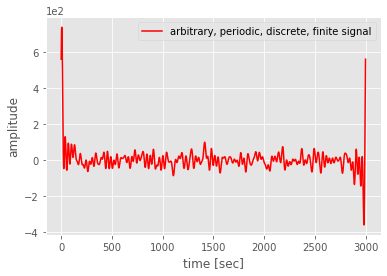Now, we can play with the signal and see what happens when we try to reconstruct it with a limited number of coefficients.
2) Run the cells 4 and 5. What do you observe?
3) Increase the number of coefficients $n$ step by step and re-run cells 4 and 5. What do you observe now? Can you explain?
4) In cell 5 uncomment the lines to make a plot which is not normalized (and comment the other two) and re-run the cell. What do you see now and can you explain it?

In :
# Cell 4: determine the first 'n' coefficients of the function using the code function of cell 1
T = 1        # period
n = 5        # number of coeffs to reconstruct
a0, a, b = fourier_series_coeff(sig, T, n)
a_ = a.astype(int)
b_ = b.astype(int)
print('coefficient a0 = ', int(a0))
print('array coefficients ak =', a_)
print('array coefficients bk =', b_)

coefficient a0 =  248
array coefficients ak = [1093 1344  846 2346 1605]
array coefficients bk = [ 252  504  507  505 2507]

In :
# Cell 5: reconstruct the function using the code in cell 2
g = series_real_coeff(a0, a, b, t, dt)

# plotting
#plt.plot(t, sig, 'r', label='original signal')          # NOT normalized
#plt.plot(t, g, 'g', label='reconstructed signal')
plt.plot(t, sig/max(sig), 'r', label='original signal')  # normalized
plt.plot(t, g/max(g), 'g', label='reconstructed signal')

plt.ticklabel_format(axis='y', style='sci', scilimits=(-1,1))
plt.xlabel('time [sec]')
plt.ylabel('amplitude')
plt.legend()
plt.show()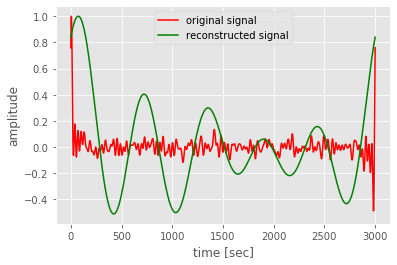### Fourier series, convergence and Gibb's phenomenon¶

As seen above the convergence of the Fourier series can be tricky due to the fact that we work with signals of finite length. To analyse this effect in a bit more detail, we define a square wave in cell 6 and try to reconstruct it in cell 7.
5) First, we use only 5 coefficients to reconstruct the wave. Describe what you see.
6) Increase the number of coefficients $n$ in cell 7 step by step and re-run the cell. What do you see now? Can you explain it?

In :
# Cell 6: define a square wave of 5 Hz
freq = 5.
npts = 500
dt_ = 0.002
length = npts * dt_
t_ = np.linspace(0, length, npts, endpoint=False)
square = signal.square(2 * np.pi * freq * t_)

plt.plot(t_, square)
plt.xlabel('time [sec]')
plt.ylabel('amplitude')
plt.xlim(0, 1.05)
plt.ylim(-1.2, 1.2)
plt.show()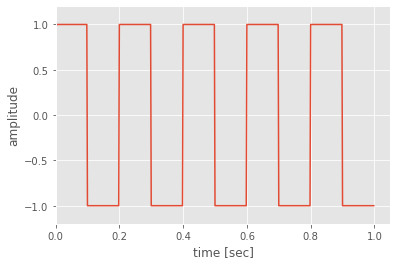In :
# Cell 7: reconstruct signal using convergence criterion
n = 5                 # number of coefficients (initial: 5)
T_ = 1/freq           # period of signal

# determine coefficients
a0 = 0
a = []
b = []
for i in range(1,n):
if (i%2 != 0):
a_ = 4/(np.pi*i)
else:
a_ = 0
a.append(a_)
b_ = (2*np.pi*i)/T_
b.append(b_)

# reconstruct signal
g = np.ones_like(t_) * a0
for k, (ak, bk) in enumerate(zip(a, b)):
g += ak * np.sin(bk*t_)

# plotting
plt.plot(t_, square, 'r', label='original signal')
plt.plot(t_, g, 'g', label='reconstructed signal')
plt.ticklabel_format(axis='y', style='sci', scilimits=(-1,1))
plt.xlabel('time [sec]')
plt.ylabel('amplitude')
#plt.ylim(-1.1,1.1)
plt.legend()
plt.show()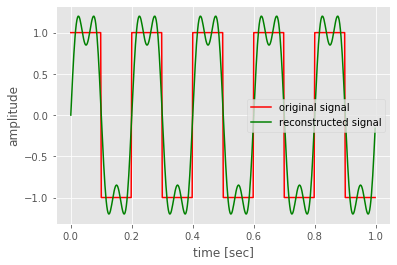### Fourier transformation¶

Let us now do the Fourier transformation of the signal created in cell 3 and have a look on the amplitude spectra. In computer science the transformation is performed as fast Fourier transformation (FFT).

7) Why do we need to taper the signal before we perform the FFT?
8) How do you interpret the plot of the amplitude spectra?
9) Which frequency contributes most to the final signal?

In :
# Cell 8: FFT of signal
# number of sample points need to be the same as in cell 3
print('samp =',samp,' Need to be the same as in cell 3.')
# number of sample points need to be the same as in cell 3
print('T =',T,' Need to be the same as in cell 3.')
# percentage of taper applied to signal (initial: 0.1)
taper_percentage = 0.1
taper = cosine_taper(samp,taper_percentage)

sig_ = sig * taper
Fsig = np.fft.rfft(sig_, n=samp)

# prepare plotting
xf = np.linspace(0.0, 1.0/(2.0*T), (samp/2)+1)
rcParams["figure.subplot.hspace"] = (0.8)
rcParams["figure.figsize"] = (15, 9)
rcParams["axes.labelsize"] = (15)
rcParams["axes.titlesize"] = (20)
rcParams["font.size"] = (12)

#plotting
plt.subplot(311)
plt.title('Time Domain')
plt.plot(t, sig, linewidth=1)
plt.xlabel('Time [s]')
plt.ylabel('Amplitude')

plt.subplot(312)
plt.title('Frequency Domain')
plt.plot(xf, 2.0/npts * np.abs(Fsig))
plt.xlim(0, 0.04)
plt.xlabel('Frequency [Hz]')
plt.ylabel('Amplitude')

samp = 3000  Need to be the same as in cell 3.
T = 1  Need to be the same as in cell 3.

/Users/lion/miniconda3/envs/seismo_live/lib/python3.7/site-packages/ipykernel_launcher.py:14: DeprecationWarning: object of type <class 'float'> cannot be safely interpreted as an integer.


Out:
Text(0, 0.5, 'Amplitude')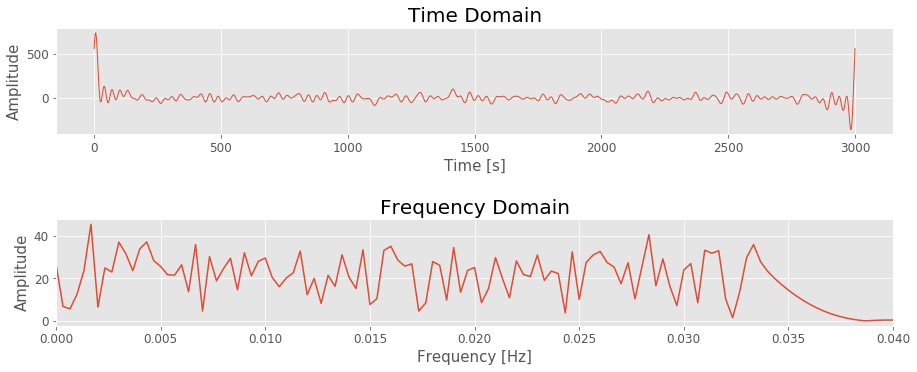1) No, you do not always see the same function. This is because the function is set up with random numbers for the weighting factors $a_k$ and $b_k$. When we re-construct a function using the Fourier series, we not only need the basis functions $\sin$ and $\cos$ but also the weighting factors. They are as important as the basis functions and give us information on how much content/amplitude the basis functions for the single summands contribute to the final Fourier sum.

2) The reconstructed signal (green) is not very similar to the original one (red) and has a longer period. Obviously, the original function is quite complex and cannot be described with only a handful of Fourier series coefficients.
3) The similarity between original and reconstructed signals increase. The general shape slowly, slowly converges to the original form. Therefore, the more coefficients are used, the better we can reconstruct the original function. This behaviour is called convergence.
4) When using only very few coefficients to reconstruct the signal, the absolute amplitudes are very different. When using more coefficients, in the middle part the amplitudes become more similar. This is because the total energy is "spread" over more coefficients.
Only at the beginning and at the end of the signals are there still large differences in the amplitudes. We can reason this phenomenon with the properties of the Fourier series. Per definition, the Fourier series (and therefore also the Fourier transformation) assumes signals have infinite length. Because we are working with a signal of finite length, we obtain "edge phenomena". They can be problematic (as seen in the section "convergence + Gibb's phenomenon" of this notebook) and have to be considered carefully.

5) The reconstructed function follows the general shape of the original wave but is not really square. It also shows unwanted undulations.
6) The more coefficients are used, the better the square shape can be reconstructed. However, the undulations now concentrate at the edges and get more distinct. This is called the Gibb's phenomenon and can be explained with the convergence properties of the Fourier series. The Fourier series converges pointwise, i.e. the signal needs to be continuous. In case of discontinuities, the lines are taken as average from the sample before and after the discontinuity. This we see as undulations.

7) The Fourier transformation assumes an infinite signal. Because this is not the case, the FFT annex the signal or rather wrap it around. Thus, the end of the signal is connected with its beginning. Because the last sample has a different amplitude than the first sample, we artificially introduce a discontinuity which leads to the Gibb's phenomenon. To avoid this trap, we bring the first and the last sample to the same amplitude by tapering. By convenience, we choose zero as the common amplitude.
8) The peaks at the different frequencies give us the amount of amplitude this frequency contributes to the final signal. We can easily see which frequencies are included and which not.
9) This answer cannot be given in an absolute way because the signal in cell 3 is made with random weighting factors and therefore the answer changes each time the cell is executed. Relatively speaking, these are the frequencies with the highest peaks/amplitudes.